Coordinate Geometry, Basic Introduction, Practice ProblemsCoordinate Geometry, Basic Introduction, Practice Problems

This video tutorial provides a basic introduction into coordinate geometry. It contains plenty of examples and practice problems.

Here is a list of topics:
1. How to identify the missing coordinate of a right triangle
2. How to calculate the area of a right triangle given the 3 vertices
3. How to identify the coordinates of the center of a circle given the endpoints of a diameter
4. How to calculate the area of a circle
5. Circumference of a circle
6. Writing the standard equation of a circle
7. Midpoint Formula
8. Distance formula between two points
9. How to calculate the slope of a line given two points
10. Writing linear equations in slope intercept form
11. Point slope formula for linear equations
12. Slope of perpendicular lines
13. Standard form of linear equations
14. Writing the equation of the tangent line
15. How to find the x intercept and y intercept of a linear equation
16. Calculating the area of the shaded region
17. How to calculate the distance between two points in 3 dimensions
18. How to plot points in 3d
19. How to find the distance between a point and a line
20. How to calculate the area of the shaded of a circle inscribed in a square
21. Area of an equilateral triangle
22. 30 60 90 Triangles – Special right triangles
23. Writing the equation of a median
24. Writing the equation of an altitude
25. Writing the equation of the perpendicular bisector

Geometry Playlist:

https://www.patreon.com/MathScienceTutor

algebra tutors,coordinate geometry,basic introduction,problems,geometry,practice problems,area of a circle,circumference of a circle,midpoint formula,circles,distance formula,slope intercept form,slope,writing linear equations,perpendicular lines,area,tangent lines,perpendicular bisectors,altitude,median,30 60 90 triangle,area of an equilateral triangle,x intercept,y intercept

algebratutors.org

45 Responses to “Coordinate Geometry, Basic Introduction, Practice Problems”

1.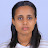Judi Semagne says:

I'm from Ethiopia thank u man

2.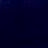J says:

thank you

3.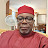Coachman Collins says:

so clear n well narrated

4.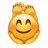Jefferey says:

this is uncannily easy to understand

5.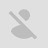Olivia Wilks 09 says:

This helped so much thank you

6.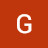Gideon A. says:

Great content but would help if you could explain the reasoning behind the formulas before using them rather than just assuming the formulas must already be known. Not always the case where one remembers these formulas nor memorize them by heart.

7.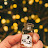Hayat Jemal says:

Thank you

8.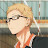Saad Shoaib says:

17:50 just marking for myself

9.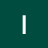Ismail Pearls says:

Is your channel far from average

10.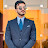علي - says:

find the line L passes through the point (1,2) and parallel the line L' x+2y=3

11.Dave Callangan says:

TYSM you're like a God lol

12.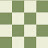Pancake Fist says:

Question 6 is wrong, I did it using algebra and got 4.3, I then did it with graphing technology, and I got the same answer. The formula you used is new to me, and I think its actually just an approximation,

13.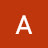Afroz says:

Tomorrow, I have a test. If, I get bad grades, they will call my mum. She will yell at me. Plus, this test's grades are getting added in my Math Exam.

14.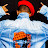Millan Ian says:

that's so great….

15.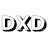DxD says:

oh gosh, got maths tomorrow

16.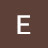Erjo Andres says:

Thanks.

17.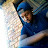Lebogang Mncedisi says:

Bro u sometimes too fast

18.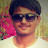Pushkar Bora says:

8th question incomplete hai

19.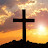Nico says:

06:14 – Simplified to (X²-16)+(y²-36) -> X² + Y² – 52?

20.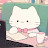Fatima Manie says:

Who's watching this in 2020?👀…lol and writing in the next 2hr45mins👀😭

21.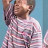tysinner says:

i'm writing my finals paper tomorrow 👍

22.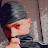Brayton says:

Writing maths final in about a week time 👀🤧😥 still got alot to revise and study .But I have to push 🙌

23.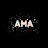AC says:

2020 igcse🤓🚮

24.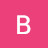Bilal Muhammad says:

25.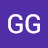GG says:

Me: *hearing the "in this video intro"
Also Me: It`s going to be a good tutorial

26.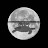Mandakhbayar .M says:

can someone explain why did he say the hyponeus was 6 square long in the first few minutes? its supposed to be 5 using the pythagorean theorem. you can literally draw a triangle with the sides 3,4 and still measure that the other side is 5. ?

27.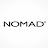Its Nomadzz says:

What if They don’t give you the point for B. Help this was on my sat!!! How do u find the y coordinate of the midpoint A and C. 25:46

28.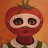Fair Garth says:

I love it when you present the math problem at first and I'm like I have no clue how to solve this in the slightest. Then you show us how to solve it and I'm like wow that was common sense x)

29.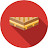josef says:

30.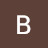georgeyf • says:

22:25 that was so confusing lol, took me awhile to get that u are using sin rule

31.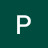10K subs with no videos says:

Why are you here on Earth? This planet really doesn't deserve a god like you.

32.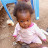Sheku Koroma says:

it's so fantastic

33.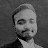M.F. Siddique says:

Any Prisoners from Quarantine?

34.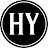하효현 says:

In 30:09 I want to make it clearly that y=mx+b is slope intercept formula but not point slope formula, y-y1=m(x-x1), because JG just say it the wrong name of formula.

35.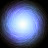Alexius Czar P. Vergara says:

Why do we write the formulas if we know we can just calculate them. Why do professors waste time writing in chalk lol

36.Alexius Czar P. Vergara says:

This is so fun and all until i realize, i havent even learned this and im almost grade 10!

37.Luke Saadeh says:

I love how he has an ad in this that would make his channel redundant

38.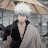リンブAaron says:

From Nepal, it is great so i could revise for my examination(grade X)

39.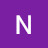run126 Reddy says:

Thank you, good explanation
From india

40.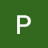Party Tomorrow says:

we dont need anymore isis future recruits

41.Party Tomorrow says:

but some news helps

42.Party Tomorrow says:

never talk show or say anything to the americans anything will make or break our negotiations keep them in the dark

43.Party Tomorrow says:

its basically drunk with power vs sane individuals who just need stability and peace to prosper

44.Party Tomorrow says:

i need your full cooperation and we need to make some surgeries happen

45.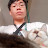zyh says:

I got it… Then I got lost